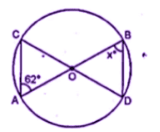Guru

# If O is the centre of the circle, find the value of x in each of the following figures (using the given information):

• 0

This is an important and exam oriented question from Chapter name- circles
Topic – Angle properties of circles
Chapter number- 15

In this question we have figure of circles with constructions and we have to find the value of x using basic properties of circles.
ICSE Avichal publication
Understanding ICSE Mathematics
Question 2

Share

1. ∠ACD = ∠ABD

(angles in the same segment)

∠ACD = xo

Now in triangle OAC,

OA = OC

(radii of the same circle)∠ACO = ∠AOC

(opposite angles of equal sides)

Therefore, xo = 62o

• 0